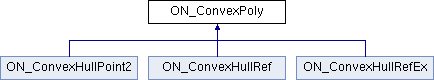Rhino C++ API  7.26
ON_ConvexPoly Class Referenceabstract

#include <opennurbs_convex_poly.h>

Inheritance diagram for ON_ConvexPoly:## Public Member Functions

virtual ~ON_ConvexPoly ()

virtual int Count () const =0

ON_3dPoint Evaluate (ON_4dex dex, ON_4dPoint B) const

bool GetClosestPoint (const ON_ConvexPoly &BHull, ON_4dex &Adex, ON_4dex &Bdex, ON_4dPoint &bary, double maximum_distance=ON_DBL_MAX) const

bool GetClosestPoint (ON_3dPoint P0, ON_4dex &dex, ON_4dPoint &bary, double maximum_distance=ON_DBL_MAX) const

bool GetClosestPointSeeded (const ON_ConvexPoly &BHull, ON_4dex &Adex, ON_4dex &Bdex, ON_4dPoint &bary, double maximum_distance=ON_DBL_MAX) const

bool GetClosestPointSeeded (ON_3dPoint P0, ON_4dex &dex, ON_4dPoint &Bary, double maximum_distance=ON_DBL_MAX) const

bool IsValid4Dex (const ON_4dex &D) const

virtual double MaximumCoordinate () const =0

ON_3dPoint Support (ON_3dVector W, int i0=0) const

virtual int SupportIndex (ON_3dVector W, int i0=0) const =0

virtual ON_3dVector Vertex (int i) const =0

## Static Public Member Functions

static bool IsValid4DexN (const ON_4dex &D, int n)

static bool Standardize (ON_4dex &D, ON_4dPoint &B)

## Detailed Description

This is a base class for a convex polytope in 3d space, i.e. the convex hull of a finite set of points called verticies.

This is the base type in the implementation of the GJK algorithm ClosestPoint(ON_ConvexPoly& A, ON_ConvexPoly& B, ...)

## ◆ ~ON_ConvexPoly()

 virtual ON_ConvexPoly::~ON_ConvexPoly ( )
inlinevirtual

## ◆ Count()

 virtual int ON_ConvexPoly::Count ( ) const
pure virtual

Returns: Number of verticies >=0

Implemented in ON_ConvexHullPoint2, and ON_ConvexHullRef.

## ◆ Evaluate()

 ON_3dPoint ON_ConvexPoly::Evaluate ( ON_4dex dex, ON_4dPoint B ) const
inline

Description: Points in a Convex Polytope are parameterized , not necessaily uniquely, by an ON_4dex of vertex indicies and a 4d barycentric point B Evaluate(Ind, B ) = Sum_{i=0,..,3} Vertex(Ind[i])*B[i], where the sum is taken over i such that Ind[i]>=0 If B is a barycentric coordinte B[i]>=0 and B + B + B + B = 1.0 then Evaluate( Ind, B) is a point in the convex polytope

## ◆ GetClosestPoint() [1/2]

 bool ON_ConvexPoly::GetClosestPoint ( const ON_ConvexPoly & BHull, ON_4dex & Adex, ON_4dex & Bdex, ON_4dPoint & bary, double maximum_distance = ON_DBL_MAX ) const

Description: Computes a pair of points on *this and BHull that achieve the minimum distance between the two convex polytopes. Parameters: BHull - [in] the other convex polytope adex, bdex -[out] Evaluate(adex,bary) is the closest point on this polyhedron bary - [out] BHull.Evaluate(bdex,bary) is the closest point on BHull. maximum_distance - [in ] optional upper bound on distance

Returns: Returns true if a closest points are found and they are within optional maximum_distance bound;

Details: Setting maximum_distance can speedup the calculation in cases where dist(*this, BHull)>maximum_distance.

## ◆ GetClosestPoint() [2/2]

 bool ON_ConvexPoly::GetClosestPoint ( ON_3dPoint P0, ON_4dex & dex, ON_4dPoint & bary, double maximum_distance = ON_DBL_MAX ) const

Description: Computes the closest point on this convex polytope from a point P0. Parameters: P0 - [in] Base Point for closest point dex -[out] bary - [out] Evaluate(dex,bary) is the closest point on this polyhedran maximum_distance - [in ] optional upper bound on distance

Returns: Returns true if a closest point is found and it is within optional maximum_distance bound;

Details: Setting maximum_distance can speedup the calculation in cases where dist(P0, *this)>maximum_distance.

## ◆ GetClosestPointSeeded() [1/2]

 bool ON_ConvexPoly::GetClosestPointSeeded ( const ON_ConvexPoly & BHull, ON_4dex & Adex, ON_4dex & Bdex, ON_4dPoint & bary, double maximum_distance = ON_DBL_MAX ) const

Expert version of GetClosestPoint.
Adex and Bdex are used at input to seed search algorithm. the points of this-Bhull singled out by Adex and Bdex must define a nondegenerate simplex

## ◆ GetClosestPointSeeded() [2/2]

 bool ON_ConvexPoly::GetClosestPointSeeded ( ON_3dPoint P0, ON_4dex & dex, ON_4dPoint & Bary, double maximum_distance = ON_DBL_MAX ) const

Expert version of GetClosestPoint.
dex is used at input to seed search algorithm. the points of *this singled out by dex must define a nondegenerate simplex

## ◆ IsValid4Dex()

 bool ON_ConvexPoly::IsValid4Dex ( const ON_4dex & D ) const
inline

## ◆ IsValid4DexN()

 static bool ON_ConvexPoly::IsValid4DexN ( const ON_4dex & D, int n )
inlinestatic

Returns: true if d[i]<n for i=0..3 a valid ON_4dex for a point in a ON_ConvexPolyBase with Count()=n

## ◆ MaximumCoordinate()

 virtual double ON_ConvexPoly::MaximumCoordinate ( ) const
pure virtual

Description: This is a bound on the collection of verticies. Vertex(i).MaximumCoordinate()<= MaximumCoordinate() for all i

Implemented in ON_ConvexHullPoint2, and ON_ConvexHullRef.

## ◆ Standardize()

 static bool ON_ConvexPoly::Standardize ( ON_4dex & D, ON_4dPoint & B )
static

Description: A point represented by a ON_4dex D and a barycentric coordinate B can be put in a standard form so that non-negative elements of D are unique and corresponding coordinates are positive. Furthemore, the non-negative indicies are all listed before the unset ( neagative ) values

## ◆ Support()

 ON_3dPoint ON_ConvexPoly::Support ( ON_3dVector W, int i0 = 0 ) const
inline

Description: Let K be this ON_ConvexPoly then for a non-zero vector W the support Support(W) are point in K defined by arg max x * W x \in K This method returns one of these points in Support(W). i0 is an optional initial index seed value. It may provide a performance enhancement toward finding a minimizer.

## ◆ SupportIndex()

 virtual int ON_ConvexPoly::SupportIndex ( ON_3dVector W, int i0 = 0 ) const
pure virtual

Description: For any vector W there is a vetex that is Support(W) SupportIndex( W, i0) returns a vertex index for a vertex that is the support. Veretx( K.SupportIndex( W )) = K.Support(W );

Implemented in ON_ConvexHullPoint2, and ON_ConvexHullRef.

## ◆ Vertex()

 virtual ON_3dVector ON_ConvexPoly::Vertex ( int i ) const
pure virtual

Returns: Vertex[i] for i=0,...,Count()-1

Implemented in ON_ConvexHullPoint2, and ON_ConvexHullRef.